# MultiProduct

Product of Reals: y = u*u* ... *u[n]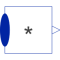# Information

This information is part of the Modelica Standard Library maintained by the Modelica Association.

This blocks computes the scalar Real output "y" as product of the elements of the Real input signal vector u:

```y = u*u* ... *u[N];
```

The input connector is a vector of Real input signals. When a connection line is drawn, the dimension of the input vector is enlarged by one and the connection is automatically connected to this new free index (thanks to the connectorSizing annotation).

The usage is demonstrated, e.g., in example Modelica.Blocks.Examples.RealNetwork1.

If no connection to the input connector "u" is present, the output is set to zero: y=0.

# Parameters (2)

significantDigits Value: 3 Type: Integer Description: Number of significant digits to be shown in dynamic diagram layer for y Value: 0 Type: Integer Description: Number of input connections

# Connectors (2)

u y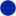Type: RealVectorInput[nu]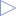Type: RealOutput

# Used in Examples (3)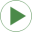RealNetwork1 Modelica.Blocks.Examples Demonstrates the usage of blocks from Modelica.Blocks.MathUniformNoiseProperties Modelica.Blocks.Examples.NoiseExamples Demonstrates the computation of properties for uniformally distributed noiseNormalNoiseProperties Modelica.Blocks.Examples.NoiseExamples Demonstrates the computation of properties for normally distributed noise

# Used in Components (2)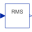RootMeanSquare Modelica.Blocks.Math Calculate root mean square over period 1/f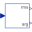Harmonic Modelica.Blocks.Math Calculate harmonic over period 1/f